# Logic Gates

## Overview

Computer circuits are built out of simple transistors, but computer designers don't often deal with transistors directly. Instead, they use higher level structures built from transistors. The simplest of these are the gates.

Logic gates implement simple boolean functions with transistors.

## Seven Logic Gates

Below are truth tables and the diagrams used for each of the seven most common logic gates.

• and

 a b a and b 0 0 0 0 1 0 1 0 0 1 1 1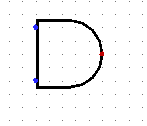• or

 a b a or b 0 0 0 0 1 1 1 0 1 1 1 1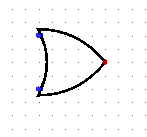• not

 a not a 0 1 1 0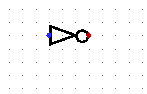• nor

 a b a nor b 0 0 1 0 1 0 1 0 0 1 1 0• nand

 a b a nand b 0 0 1 0 1 1 1 0 1 1 1 0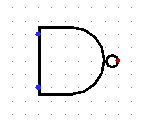• xor

 a b a xor b 0 0 0 0 1 1 1 0 1 1 1 0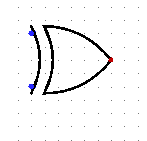• xnor

 a b a xnor b 0 0 1 0 1 0 1 0 0 1 1 1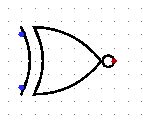How could each of these be implemented with transistors?





## Example: A Multiplexer

One simple component we can make out of logic gates is a multiplexer which takes two inputs, a and b, and a third input, s which selects between them. A truth table for a multiplexer is given below:

 a b s Output 0 0 0 0 0 0 1 0 0 1 0 0 0 1 1 1 1 0 0 1 1 0 1 0 1 1 0 1 1 1 1 1

The s input "selects" which of a or b pass through. When 0, a is the result; when 1, b is the result.

How could we build this with our gates?





## Example: A Decoder

Another simple circuit we can build is a decoder which takes a number of inputs which represent one value, and split it into one signal for each possible value. For example, we could make a two-to-four decoder which takes a two bit input, and which has four outputs, one for each of the possible two bit values.

A truth table for the two-to-four decoder is given as:

 i0 i1 o0 o1 o2 o3 0 0 1 0 0 0 0 1 0 1 0 0 1 0 0 0 1 0 1 1 0 0 0 1

How could we build this with our gates?





## Example: Comparator

Consider a circuit that has 4 inputs, representing two 2-bit values. How could we design the circuit such that it outputs a 1 when the two values are equal, and a 0 when the two values are different?





Now how could we add two more outputs, one representing that the first number is less than the other, and one representing that it is greater?





## Universal Gates

We actually don't need all seven logic gates to build circuits. We can in fact build all of the other gates using just AND and NOT, or just OR and NOT

How could we build an OR gate using AND and NOT gates?





How could we build an AND gate using OR and NOT gates?





There are actually two gates which are "universal" in that they alone can be used to build any of the other gates, and in fact any logical function whatsoever. Those two gates are NAND and NOR.

The construction of the other gates out of NAND and NOR is slightly more complicated than the two examples above, but the constructions are given in the Wikipedia articles on NAND Logic and NOR Logic.

## NAND vs. NOR

Because NAND and NOR are universal, they are often used to implement entire circuits. Either type of gate could be used to build any piece of circuitry we could want.

NAND gates are actually preferred because they can be made to be faster than NOR gates. A NAND gate can be constructed from two P-type transistors in parallel: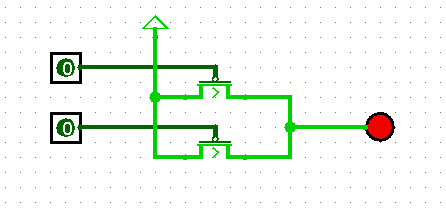A NOR gate can be constructed from two P-type transistors in sequence: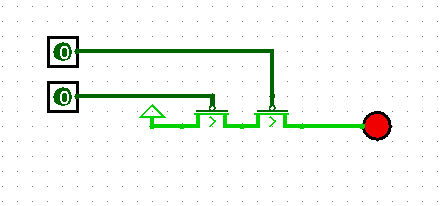NAND gates switch faster than NOR gates, because the time for the entire gate is the time for one transistor to switch. With the NOR gate, the time taken is the time for two transistors to switch, one after the other.

NAND gates are the most widely used because they are universal, only require two transistors, and switch quickly.

However, designers don't need to think in terms of NAND gates directly. As we will see, there are tools which can take logical expressions and "compile" them into circuits which use only NAND.